# Theorems of Perpendicular and Parallel Axes

#### notes

Theorem of perpendicular axis:

Perpendicular Axis Theorem: The moment of inertia of a planar body (lamina) about an axis perpendicular to its plane is equal to the sum of its moments of inertia about two perpendicular axes concurrent with perpendicular axis and lying in the plane of the body.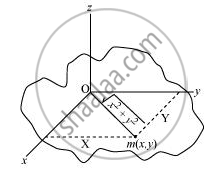Moment of inertia about x-axis, Ix = mx2

Moment of inertia about y-axis, Iy = my2

Moment of inertia about z-axis, "I"_z= m(sqrt(x2+y2))^2

I_x + I_y = mx^2 + my^2

= m(x^2 + y^2)

=m(sqrt(x2+y2))

Ix+Iy=Iz

Hence the theorem is proved

Applicable only to planar bodies.

### Theorem of parallel axis:

Parallel Axis Theorem: The moment of inertia of a body about any axis is equal to the sum of the moment of inertia of the body about a parallel axis passing through its centre of mass and the product of its mass and the square of the distance between the two parallel axes.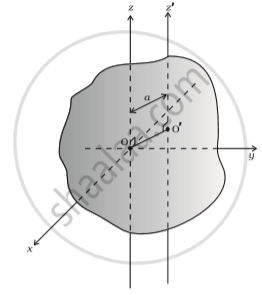This theorem is applicable to a body of any shape.

Example: Given the moment of inertia of a disc of mass M and radius R about any of its diameters to be "MR"^2/4, find its moment of inertia about an axis normal to the disc and passing through a point on its edge

Solution: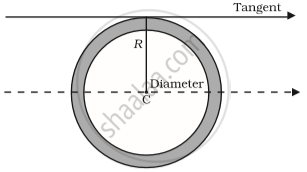We can apply Perpendicular axis theorem here on x axis & y axis and get I’, moment of inertia in z axis.
"l"_"z" = "l"_"x" + "l"_"y", now as because of symmetry Ix & Iy are same so Iz = I’ = 2I = ("Mr"^2)/2

Now we can apply parallel axis theorem to find I’’.
I'' = I' + "MR"^2 = 3/2("MR"^2)

Moment of Inertia:

 Z Body Axis Figure I 1 Thin circular ring, radius R Perpendicular to plane, at centre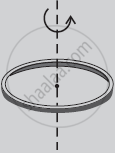MR2 2 Thin circular ring, radius R Diameter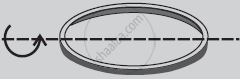"MR"^2/2 3 Thin rod, length L Perpendicular to rod, at mid point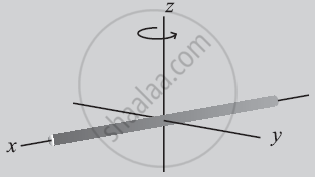"ML"^2/12 4 Circular disc, radius R Perpendicular to disc at centre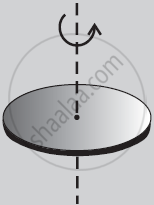"MR"^2/2 5 Circular disc, radius R Diameter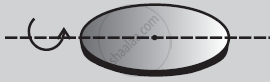"MR"^2/4 6 Hollow cylinder, radius R Axis of cylinder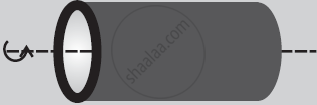MR2 7 Solid cylinder, radius R Axis of cylinder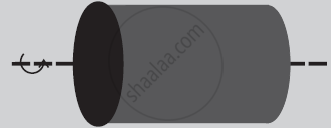"MR"^2/2 8 Solid sphere, radius R Diameter2 "MR"^2/5

#### description

• Theorem of Perpendicular Axes
• Theorem of Parallel Axes
• Application of perpendicular and parallel axes theorem on different regular bodies
If you would like to contribute notes or other learning material, please submit them using the button below.

#### Video Tutorials

We have provided more than 1 series of video tutorials for some topics to help you get a better understanding of the topic.

Series 1

Series 2

### Shaalaa.com

Principle of parallel and perpendicular axes [00:15:57]
S
0%